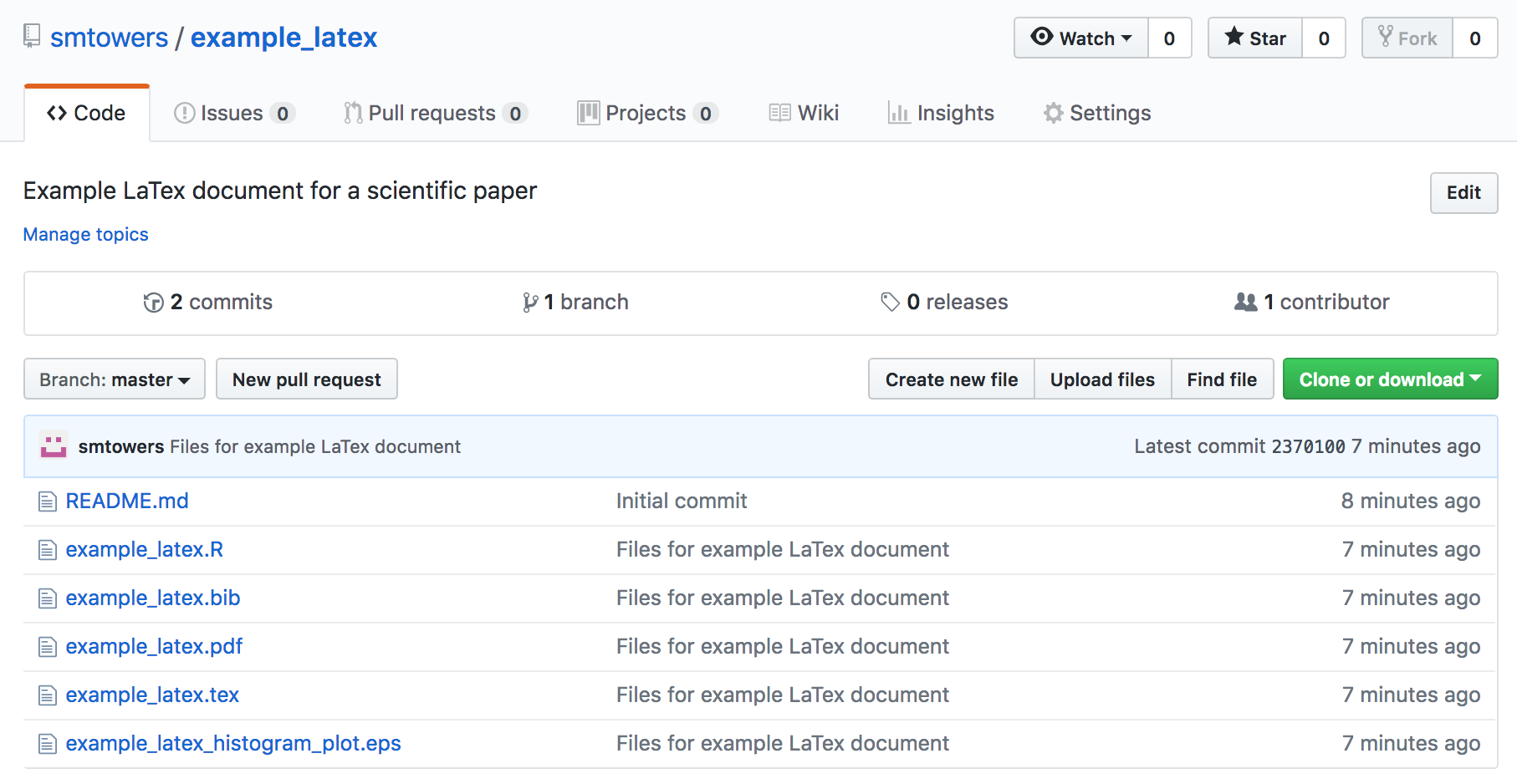# Testing if one model fits the data significantly better than another model

When doing Least Squares or likelihood fits to data, sometimes we would like to compare two models with competing hypotheses. In this module, we will discuss the statistical methods that can be used to determine if one model is significantly statistically favoured over another.

# Graphical Monte Carlo method: choosing ranges over which to sample parameters

In this module we will discuss how to choose ranges over which to sample parameters using the graphical Monte Carlo method for fitting the parameters of a mathematical model to data.  We will also discuss the importance of using the Normal negative log-likelihood statistic (equivalent to Least Squares) when doing Least Squares fitting, rather than the Least Squares statistic itself.

# Graphical Monte Carlo parameter opimisation: Uniform random sampling

In this module, we will discuss the graphical Monte Carlo parameter optimisation procedure using Uniform random sampling of the parameter hypotheses, and compare and contrast this method with the graphical Latin hypercube method.

# Running R in batch with ASU high performance computing resources

In this module, intended for students at ASU, we discuss how to use ASU high performance computing resources to run an R script on many CPU’s simultaneously.

# Contagion models with non-exponentially distributed sojourn times in the infectious state

Compartmental models of infectious disease transmission inherently assume that the time spent (“sojourn time”) in the infectious state is Exponentially distributed.  As we will discuss in this module, this is a highly unrealistic assumption.  We will show that the “linear chain rule” can be used to incorporate more realistic probability distributions for state sojourn times into compartmental mathematical models.

# Example LaTex and BibTex documents

In this module, I provide an example LaTex document that cites references within a BibTex file, and also includes examples of how to include equations, figures, and tables.

The files for this worked example can be found in my GitHub repository https://github.com/smtowers/example_latexThe repository contains the main LaTex document example_latex.tex, along with the bibtex file example_latex.bib.  In order to compile the document, you also need to download the example_latex_histogram_plot.eps, which is the figure included in the file.  To compile the document, run LaTex once, then BibTex, then LaTex twice (which should resolve all references).

This should produce the file example_latex.pdf

Note that the encapsulated postscript (EPS) figure for the paper was produced with the R script example_latex.R  (you need to install the R extrafont library before running the script) The R script also shows you how to automatically output results from your analysis code that can be included as \newcommands in your latex file that allow you to copy and paste the results to your LaTex file so that reference those results in the text of your paper without having to manually transcribe numbers (which can lead to unnecessary transcription errors).

# Data and R code repositories in GitHub

GitHub is a web-based version-control and collaboration platform for software developers.

Git, an open source code management system, is used to store the source code for a project and track the complete history of all changes to that code. It allows developers to collaborate on a project more effectively by providing tools for managing possibly conflicting changes from multiple developers. GitHub allows developers to change, adapt and improve software from its public repositories for free.  Repositories can have multiple collaborators and can be either public or private.

# Visual analytics with R Shiny

In this module, students will learn about the rapidly growing field of visual analytics, and learn how to create their own online visual analytics applications using the R Shiny package.

# Computational and statistical methods for mathematical biologists and epidemiologists.

Objectives:

This course is meant to provide students in applied mathematics with the broad skill-set needed to optimize the parameters of dynamical mathematical models to relevant biological or epidemic data. The course will almost entirely be based on material posted on this website. Continue reading

# Predatory journals and conferences

In this module, we’ll briefly discuss what “predatory” journals and conferences are, the dangers they pose to early career researchers, and how to recognise and avoid them (and also how to choose reputable journals and conferences)

# Making your own R library package

In this module, we’ll discuss how to make your own R library package, and how to upload it to the R CRAN repository.

# Negative Binomial likelihood fits for overdispersed count data

In this module, students will become familiar with Negative Binomial likelihood fits for over-dispersed count data.

# Kolmogorov-Smirnov test

In this module students will become familiar with the Kolmogorov-Smirnov non-parametric test for equivalence of distributions

# Numerical methods for propagation of uncertainties

In this module we will discuss numerical methods that can be used to calculate the 95% CI on data that has been transformed by some function, if one knows the probability distribution underlying the stochasticity of the original data.

# Least Squares fitting is equivalent to homoskedastic Normal likelihood fitting

In this module, students will learn how the Least Squares fit statistic can be expressed as a likelihood.

# Model validation methods

In this module, students will become familiar with the importance of, and methods for, model validation.

# Population standardized regression methods

In this module, students will become familiar with population standardized regression methods when working with data that is expressed as per-capita rates

# Logistic (Binomial) regression

In this module, students will become familiar with logistic (Binomial) regression for data that either consists of 1′s and 0′s (“yes” and “no”), or fractions that represent the number of successes out of n trials.  We focus on the R glm() method for logistic linear regression. Continue reading